# Probability Distributions

A LevelAQAEdexcelOCR

## Probability Distributions

Random variables are variables that take different values with different probabilities. Probability distributions describe their behaviour. The cumulative distribution function is a function formed from the probability distribution, condensing all of the information about a random variable into a useful form.

A LevelAQAEdexcelOCR

## Random Variables

A random variable, usually denoted with a capital letter such as $X$, is a variable that takes different values at random with different probabilities. The different values it can take are usually denoted with lowercase letters such as $x$.

Example: $X$ takes the value of the score on a fair six-sided die. This means that $\mathbb{P}(X=1)=\dfrac{1}{6}$, $\mathbb{P}(X=2)=\dfrac{1}{6}$, etc. The possible values for $X$ are $x=1,2,3,4,5,6$

A discrete random variable can only take a certain number of different values. The example above is a discrete random variable as there are only $6$ values $X$ can take.

A LevelAQAEdexcelOCR

## Probability Distributions and Probability Functions

A probability distribution for a discrete random variable is a table showing all of the possible values for $X$ and their probabilities. The dice example would give: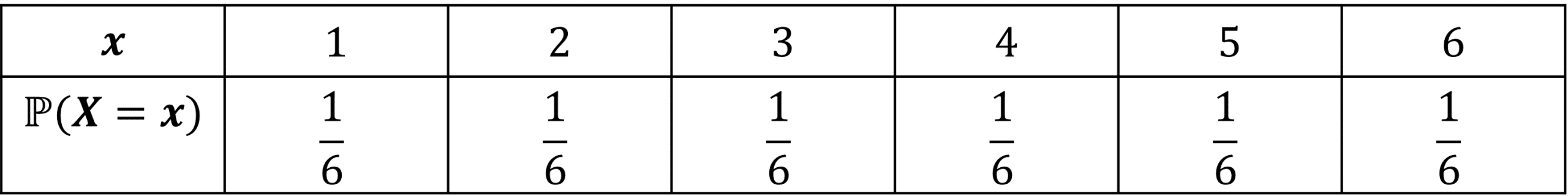Note: The probabilities for a random variable must add to 1:

$\sum_{x}\mathbb{P}(X=x)=1$

We can define the probability function:

$f(x)=\mathbb{P}(X=x)$

Finally, the cumulative distribution function is a running total of the probability function:

$F(x)=\sum_{y\leq x}f(y)=\mathbb{P}(X\leq x)$

A LevelAQAEdexcelOCR
A LevelAQAEdexcelOCR

## Example 1: Creating a Probability Distribution

Create a probability distribution for the sum of two dice rolls.

[4 marks]

Step 1: Create a table of every possible outcome. Note that all of these outcomes are equally likely.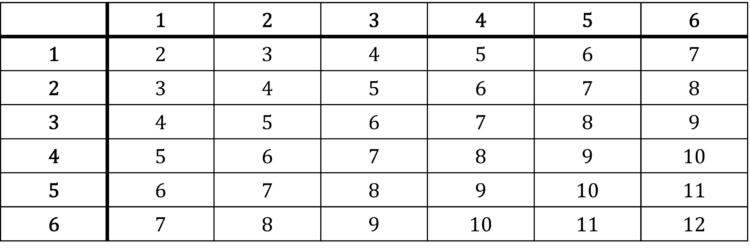There are thirty-six equally likely outcomes. One of these gives $2$, two of these give $3$, three of these give $4$, four of these give $5$, five of these give $6$, six of these give $7$, five of these give $8$, four of these give $9$, three of these give $10$, two of these give $11$ and one of these gives $12$. So the probability distribution looks like this: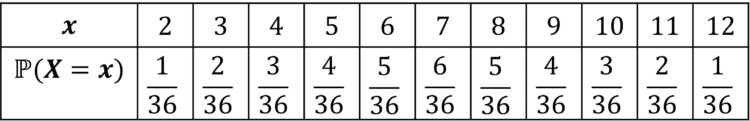And after simplifying we have our final answer: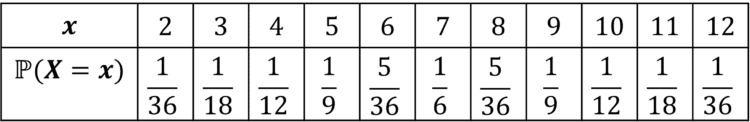A LevelAQAEdexcelOCR

## Example 2: Probability Function

From the following probability distribution, determine the probability function and the cumulative distribution function.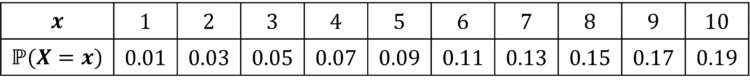[4 marks]

The probability function is defined by $f(x)=\mathbb{P}(X=x)$, so we need a function that satisfies $f(1)=0.01$, $f(2)=0.03$, $f(3)=0.05$, etc. Note that the function increases by $0.02$ each time, so is linear with a gradient of $0.02$. Hence, we might try $f(x)=0.02x+c$. Subbing in $f(1)=0.01$ gives $c=-0.01$. Hence, the probability function is $f(x)=0.02x-0.01$

To find the cumulative distribution function, we must first add a running total row to our table.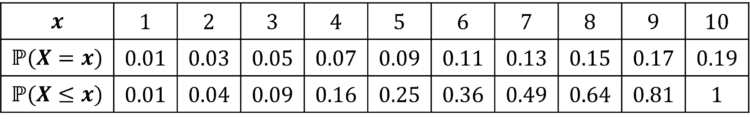The cumulative distribution function, $F(x)$, must satisfy $F(1)=0.01$, $F(2)=0.04$, $F(3)=0.09$, etc. This is satisfied by $F(x)=\dfrac{x^{2}}{100}$.

A LevelAQAEdexcelOCR

## Probability Distributions Example Questions

First create a table of all possibilities.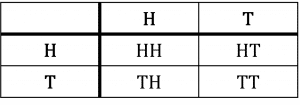There are $4$ equally likely possibilities. One gives $2$ heads, two give $1$ head and one gives no heads.

Hence, we can construct the probability distribution: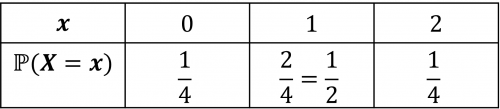a) Probabilities must add to $1$.

$0.2+n+0.3+n+0.2=1$

$2n+0.7=1$

$2n=0.3$

$n=0.15$

b) $\mathbb{P}(2\leq X\leq 4)=$

$\mathbb{P}(X=2)+\mathbb{P}(X=3)+\mathbb{P}(X=4)=$

$0.3+0.15+0.2=$

$0.65$

First establish the distribution from the probability function.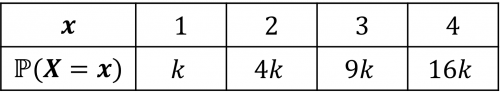Now we use the fact that probabilities add to $1$:

$k+4k+9k+16k=1$

$30k=1$

$k=\dfrac{1}{30}$

A Level

A Level

## You May Also Like...### MME Learning Portal

Online exams, practice questions and revision videos for every GCSE level 9-1 topic! No fees, no trial period, just totally free access to the UK’s best GCSE maths revision platform.

£0.00